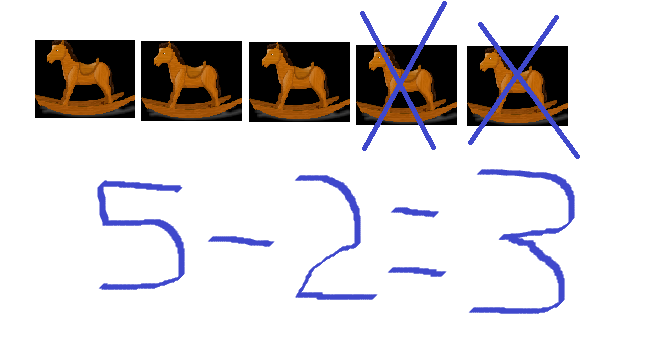# Which Method More effective for subtraction?

in #educationlast year (edited)source image of toys

Mathematics is a subject that we cannot avoid. In everyday life, mathematics will guide us to be more careful, thorough and careful. Awareness of this makes every parent need to teach a lesson to their children.

When learning mathematics we count from subtraction, subtraction, division and multiplication. This basic calculation is very important to be taught to children. Without learning, subtraction, division, and multiplication, children will find it difficult if they are presented with math problems.

### Reduction in daily life.

This time I want to write about subtraction. In everyday life subtraction is used to calculate the difference or subtraction directly.
Example:
1. Anton is 12 years old, Nia 6 years old, how old are both of them?
2. Doni has 17 candies and eats them 8. How many candies do he have left?
3. 14 - 9 =...

To calculate the three problems above, we must use subtraction.
In question number one we calculate the subtraction of 12 with 6 results 6. Question number two is calculated by subtracting 17 by 8 the result is 9. While for question number 3 the answer is 5.
For adults or who can count on it is very easy to do. However, for children who have not been able to have difficulty to answer.

### Subtraction method

Well, for that we need to provide an explanation of how to reduce subtraction. There are several methods that can be calculated to calculate the subtraction.

1. Counting down.
the method of subtraction by counting down is still mostly done by counting backwards from large numbers to small numbers.

In the example of question number 1, the child will count backwards from number 12 to number 6. Usually the hand is clenched and then starts counting 11, 10, 9, 8, 7 and 6. The result is 6.
In question number 2 the child will count backwards from 17 and stop at number 8 so that the result is 9. While question number 3 is counted from 14 to 9 and the result is 5.

This way of counting down can be done but becomes error prone. This happens because when counting down there can be a jump in the count. Counting time will also take longer because you are not used to counting down.

2. Using media
We can teach children how to calculate subtraction using media. The tools we use can be sticks, stones, or other objects that are easily available.

We use a stick as an example, so we have to prepare the number of sticks according to the number that is subtracted and then we take it according to the number used to reduce so that we can see the rest of the sticks that have not been taken. The number of sticks that have not been taken is the result of the calculation.

In example number 1 we provide 12 sticks and then take 6 sticks so that there are 6 sticks left.
In question 2 we provide 17 sticks and then take 8 so that the result is 9 and in the third question 14 sticks we take 9 remaining 5.

This method is quite helpful for children, only they have to prepare the media that will be used.

3. Counting forward
The trick is to make a fist and then count from after the small number to the large number. while opening the finger and stopping at a large number. This open finger is the result of the count.

In the first problem, we just clench our fists and count forward while opening our fingers after 6. Open the first finger while counting 7, the second finger 8 and so on until the open finger on the count of 12. Then we see the opened finger are 6.
The result of 12 - 6 is 6.

Likewise in the second question. We just need to clench our fists and then count forward after the number 8 and stop at number 17 so we get the result 9.
While the third question we count forward from after the number 9 stops at number 14 so the result is 5.

By counting forward the child does not experience significant obstacles because it is like ordinary counting. This way of calculating is much more practical and easy to learn.

Those are some ways to calculate subtraction that we can do so that it can increase understanding for children.
Thus my writing this time may be useful for all of us.

Best regard from Indonesia.
@rokhani

# Thank you for stopping by and reading my post. I hope you enjoy it. Upvote, comment, reblog and other support are highly appreciated.

Sort:

Nice topic, very educational. Looking forward for more tips from you. This is very helpful.

Nice topic,very educational! Looking forward to hear more tips from you.